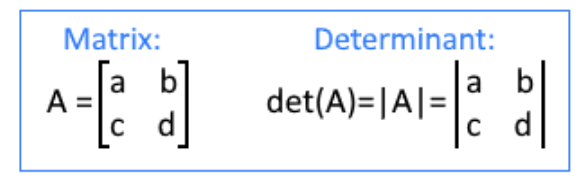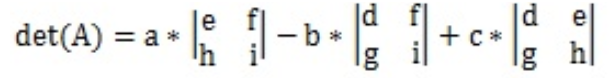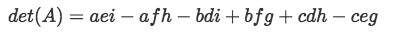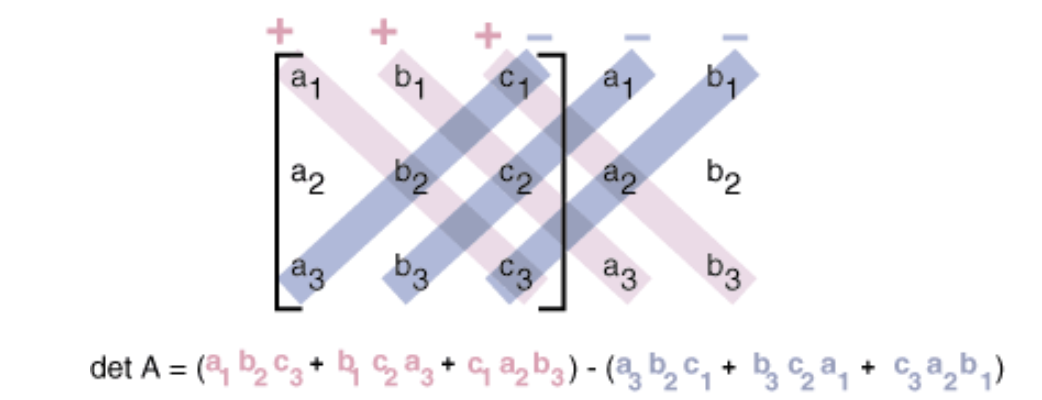# The determinant of a 3 x 3 matrix (General & Shortcut Method) - Matrices

### The determinant of a 3x3 matrix (General and Shortcut method)

As we have seen in past lessons, in order to define what is a determinant of a matrix we need to go back a to our definition of a matrix. Remember that we have learnt that a matrix is an ordered list of numbers put in a rectangular bracket. This list can also be called a rectangular array, and it provides an orderly fashion to display a "list" of information elements. If you want to review the definition of the matrix with more detail you can revisit our lesson on notation of matrices.

A matrix describes a linear transformation or linear map, which is a kind of transcription between two types of algebraic structures, such as vector fields. In that way, we can resolve systems of linear equations by representing a linear system as a matrix. The matrix representation of a linear system is made by using all of the variable coefficients found in the system, and use them as element entries to construct the rectangular array of an appropriate size augmented matrix. In such matrix, the results of each equation from the system will be placed on the right hand side of the vertical line which represents the equal sign.

Knowing that, this lesson will focus on the process for evaluating the determinant of a 3x3 matrix and the two possible methods to employ.

### What is the determinant of a matrix

By using the knowledge that a matrix is an array containing the information of a linear transformation, and that this array can be conformed by the coefficients of each variable in an equation system, we can describe the function of a determinant: a determinant will scale the linear transformation from the matrix, it will allow us to obtain the inverse of the matrix (if there is one) and it will aid in the solution of systems of linear equations by producing conditions in which we can expect certain results or characteristics from the system (depending on the determinant and the type of linear system, we can know if we may expect a unique solution, more than one solution or none at all for the system).

But there is a condition to obtain a matrix determinant, the matrix must be a square matrix in order to calculate it. Hence, the simplified definition is that the determinant is a value that can be computed from a square matrix to aid in the resolution of linear equation systems associated with such matrix. The determinant of a non square matrix does not exist, only determinants of square matrices are defined mathematically.

The determinant of a matrix can be denoted simply as det A, det(A) or |A|. This last notation comes from the notation we directly apply to the matrix we are obtaining the determinant of. In other words, we usually write down matrices and their determinants in a very similar way:Equation 1: Difference between the notation of a matrix and a determinant

Notice the difference, the matrix is written down with rectangular brackets and the determinant of the matrix has its components surrounded by two straight lines.

The lesson of today will be focused on the process to compute the determinant of a 3x3 matrix, taking approach of the matrix determinant properties, which have been briefly seen in past lessons. Remember we will look at that complete topic in a later lesson called: properties of determinants. Still, it is important to keep those properties in mind while performing the calculations of the exercises in the last section of this lesson.

### How to find the determinant of a 3x3 matrix

There are two methods for finding the determinant of a 3x3 matrix: the general method and the shortcut method. Just as the names of each of them sound, the general method is the "formal" method to use mathematically, following all the rules and producing some minor matrix determinant calculations along the way to find the final solution. While the shortcut method is more of a clever trick we can use to simplify the calculation, still being careful to not forget numbers, the order in which they have to be multiplied and some rearrangements of the elements in the matrix.

After you take a look at both methods to find the determinant of a 3x3 matrix, you can always pick whichever suits you best and use it for your studies, but remember that it is important you know both of them in case you are ever asked to compare results from them.

So, without further delay let us define the determinant of 3x3 matrix A as shown below, so we can observe how it can be calculated through both methods:

• General method

The general method to obtain the determinant of a 3x3 matrix consists of breaking down the matrix into secondary matrices of smaller dimensions in a process called "expansion of the first row". What this process does is to use the elements from the first row of the 3x3 matrix and use them as factors in a sum of multiplications where the big matrix gets redistributed.

Let us go go step by step on how to calculate the determinant of a 3x3 matrix:

1. You first take the first element of the first row and multiply it by a secondary 2x2 matrix which comes from the elements remaining in the 3x3 matrix that do not belong to the row or column to which your first selected element belongs.

Taking as a reference the 3x3 matrix determinant shown in equation 2, we construct the first part of the result of this operation by selecting the first element of the first row and column (which is constant "a"), and then multiply it by a matrix produced from the four elements which do not belong to either the row of the column in which "a" is. Multiply "a" with this secondary 2x2 matrix obtained and that is the first term of the solution.

2. The second term starts with the second element of the top row (constant "b") accompanied by a negative sign, which now will multiply a secondary 2x2 matrix which results, once more, from the four elements in the matrix which do not belong to either the column of row in which "b" is.
3. We repeat step one, but now with the third element from the top row of the matrix.

And so, the determinant of a 3x3 matrix formula for the general method is:Equation 3: Equation for determinant of a 3x3 matrix through general method

The process is called an expansion of the first row because as you can see in equation 3, all of the elements from the first row of the original 3x3 matrix remain as main factors in the expansion to be solved for. All of the 2x2 matrices in the expansion are what we call "secondary matrices", and they can be easily resolved using the equation learnt on the determinant of a 2x2 matrix lesson.

And so, taking into consideration the formula for the determinant of a square matrix with dimensions 2x2, we can see that equation 3 yields:Equation 4: Equation for determinant of a 3x3 matrix through general method (part 2)

At this point you may have noticed that finding the determinant of a matrix larger than 2x2 becomes a long ordeal, but the logic behind the process remains the same and so the difficulty is similar, the only key point is to keep track of the operations you are working through, even more with even larger matrices than a 3x3.

• Shortcut method

The determinant of a 3x3 matrix shortcut method is a clever trick which facilitates the computation of a determinant of a large matrix by directly multiplying and adding (or subtracting) all of the elements in their necessary fashion, without having to pass through the matrix expansion of the first row and without having to evaluate secondary matrices' determinants.

The whole process of how to evaluate the determinant of a 3x3 matrix using the shortcut method can be seen in the equation below:Equation 5: Shortcut method to obtain the determinant of a 3x3 matrix

Now let us explain the shortcut method clearly:

When computing the determinant of an nxn matrix (in this case a 3x3 matrix) as shown above, notice we first rewrite the matrix accompanied by a repetition of its two first columns now written outside to the right hand side.

Then, the determinant value will be the result of the subtraction between addition of products from all of the down-rightward multiplications and the down-leftward multiplications. Said clearer, there will be a total of three complete diagonals going from the top left to the bottom right, and another set of three complete diagonals going from the top right to the bottom left.

We will multiply the elements of each diagonal together, then add them with the results coming from the other diagonals. There is something to have in mind, all of the diagonals' multiplications going from top left to bottom right have an intrinsic positive sign multiplied to them, while all of the diagonals' multiplications going from top right to bottom left have an intrinsic negative sign multiplied to them, and so, when adding the results from all of the multiplications, a subtraction such as the one shown in equation 5 will result.

Although this method is simpler to perform than the general method, it is a little complicated to explain due to all of the multiplications and additions being worked at the same time, so we recommend you to use equation 5 as a guidance and pay close attention to the videos where examples of this method are being shown.

In the last section of this lesson we will work through a set of three different 3x3 matrices and their determinants, we recommend you to compared the processes for both methods to understand them better.

### Determinant of a large matrix

The process to evaluate the determinant of a matrix of greater dimensions than 3x3 follows the same logic than what we have seen so far. Using the general method on a 4x4 matrix A, where its first (top) row is conformed by the elements a, b, c and d, we evaluate the determinant of the matrix as follows:

We once more have expanded the determinant by its first row and obtained secondary matrices, which in this case happen to be 3x3 matrices which each can be expanded and broken down into 2x2 matrices. The pattern in the process repeats, you can continue working this way through even larger square matrices and it will always work, but if you are more into the shortcut method, then you are in for a treat since the method works exactly in the same manner as it does with 3x3 matrices, it just increases the amount of elements you are working with but the logic and rearrangement is exactly the same (multiplication from top left to bottom right have a positive sign, multiplications from top right corner to bottom left have an intrinsic negative sign).

Are you excited to see how the shortcut method works on larger matrices? We encourage you to try it out on your own so you can see the whole process. You can always go back and solve the same matrix using the general method and prove your result is correct.

### Exercises on evaluating the determinant of a 3x3 matrix

In the next exercises we will solve the determinant of a 3x3 matrix provided in each case with the corresponding method, and at the end we will compare the results obtained.

• Finding the determinant using the General Method
1. Having the matrix A as defined below:

Find the matrix determinant using the general method

For practical purposes we go straight to equation 4 which is a simplification of the formula for the general method shown in equation 3, and so we use equation 4 to solve all of our exercises corresponding to the general method. Then, the determinant of matrix A is:

2. Having the matrix B as defined below:

Finds its determinant using the general method:

3. Having the matrix C as defined below:

Finds its determinant using the general method:

• Finding the determinant using the Shortcut Method
1. Having the matrix A as defined below:

Find the determinant of matrix A using the shortcut method:

Following equation 5, the determinant goes as follows.

2. Having the matrix B as defined below:

Finds its determinant using the shortcut method:

3. Having the matrix C as defined below:

Finds its determinant using the shortcut method:

Notice that the matrices A, B and C provided in the both sections of exercises above are the exact same. This has been done on purpose so you can compare the results from both methods and observe how they yield the same values.

To finalize this lesson we would like to recommend you this article on how to compute determinants and this other one on the determinant of a square matrix, where you will find many more examples than the ones provided here.

We hope this lesson was fun and useful, see you in the next one!

### The determinant of a 3 x 3 matrix (General & Shortcut Method)

In this section, we will learn the two different methods in finding the determinant of a 3 x 3 matrix. Instead of memorizing the formula directly, we can use these two methods to compute the determinant. The first method is the general method. This method requires you to look at the first three entries of the matrix. For each entry, you want to multiply that entry by the determinant of a 2 x 2 matrix that is not in that entry's row or column. Note that you have to put a negative sign on the second entry. Then you add everything up, and that will be the determinant of the 3 x 3 matrix. The second method is a shortcut. Watch the video to have a clear explanation of how it works.

#### Lessons

##### The determinant of a 3 x 3 matrix Overview